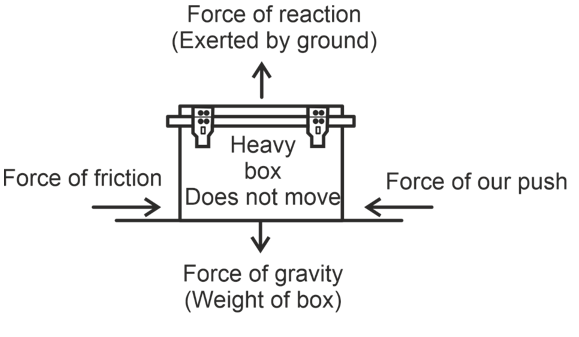# Balanced and unbalanced forces

## Balanced and unbalanced forces

Suppose a toy car can move on its wheels along east- west direction. If we push the car with our left hand, it moves towards east. If we push the car with our right hand, it will move towards west. If we push the car with our left hand towards east and with right hand towards west simultaneously, it is possible that the car will remain at rest. In this case the forces balanced each other and there is no acceleration. So if a set of forces acting on a body produces no acceleration in it, the forces are said to be balanced forces.

If it produces a non-zero acceleration, the forces are said to be unbalanced forces. If two forces balance each other, they must be in opposite direction and have equal magnitudes.

### BALANCED FORCES:

If the resultant of all the forces acting on a body is zero, the forces are called balanced forces. NCERT solutions for class 8 Science prepared by Physics Wallah will help you to solve your NCERT text book exercise.When balanced forces act on a body (here a heavy box), they do not produce any motion in it.

e.g.

• A heavy box lying on ground has four forces acting on it as shown in the figure. Since the box does not move at all, the resultant of all the forces acting on it is zero. The force of our push is balanced by the force of friction, and the force of gravity is balanced by the force of the reaction of the ground. So, the forces acting on this stationary box are an example of balanced forces.
• Similarly, in a tug of war, i.e. in rope pulling between two teams, if the resultant of forces applied by the two teams is zero, the rope does not move in either direction. The forces exerted by two teams are balanced. From the above example we also conclude that balanced forces do not change the state of rest or of motion of an object i.e. these forces cannot produce motion in a stationary body or stop a moving body. However, they can change the shape of the body. For e.g. when a spherical balloon is pressed between our two hands, its shape changes from spherical to oblong. Here, the balloon does not move, but its shape changes.
• Friction is a force which opposes the motion of a body moving over the surface of another body.

### Unbalanced Forces:

If the resultant of all the forces acting on a body is not zero, the forces are called unbalanced forces.

• A heavy box can be moved with a very strong force. This is because in that case the force of push will become greater than the opposing force of friction. An unbalanced force will then act on the heavy box and make it move.
• A ball rolling on the ground stops after some time because an unbalanced force of friction acts on it.
• When we stop pedaling, the bicycle begins to slow down. This is again because of the unbalanced friction force acting opposite to the direction of motion.

From the above examples, we also conclude that unbalanced forces can change the state of rest or state of uniform motion or the direction of the body or the size and shape of the body.

An unbalanced force is needed to move a body from state of rest but no such force is required to maintain uniform motion of the body.